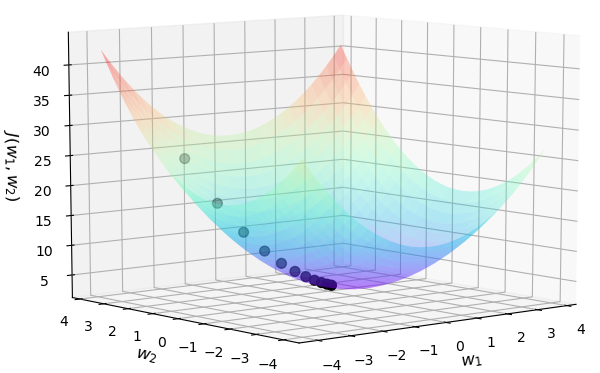### 1 引言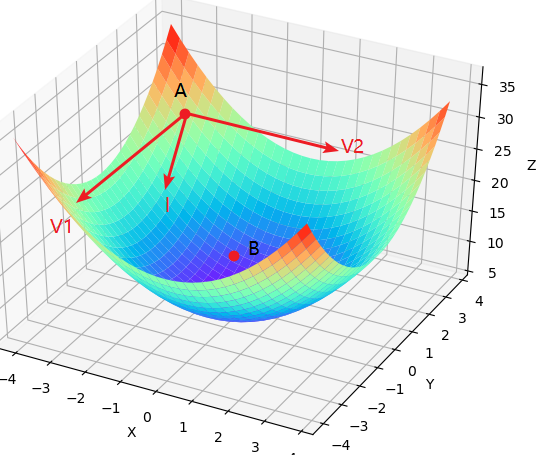### 2 梯度下降算法

#### 2.1 方向导数与梯度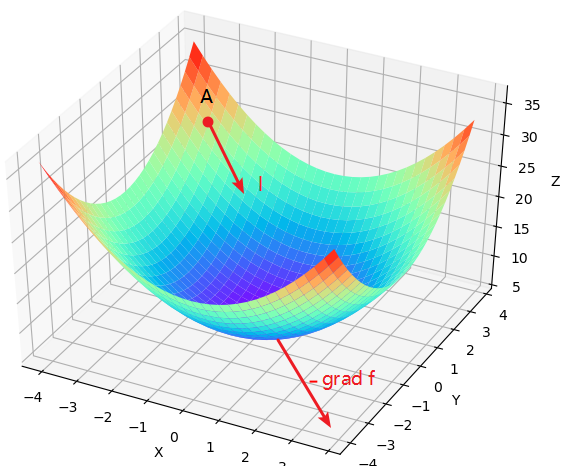#### 2.2 梯度下降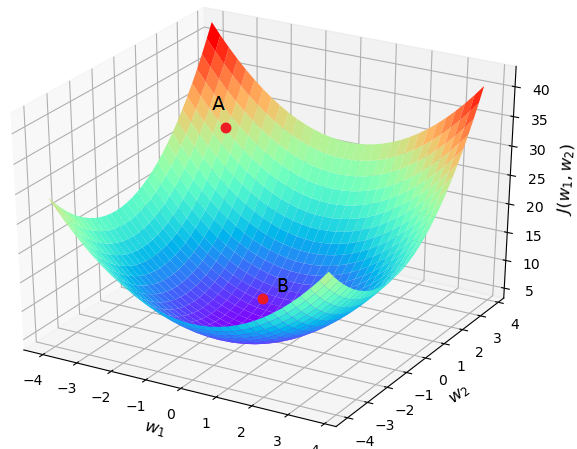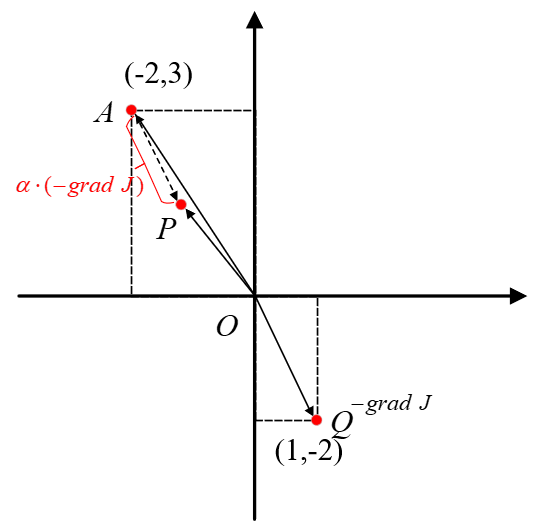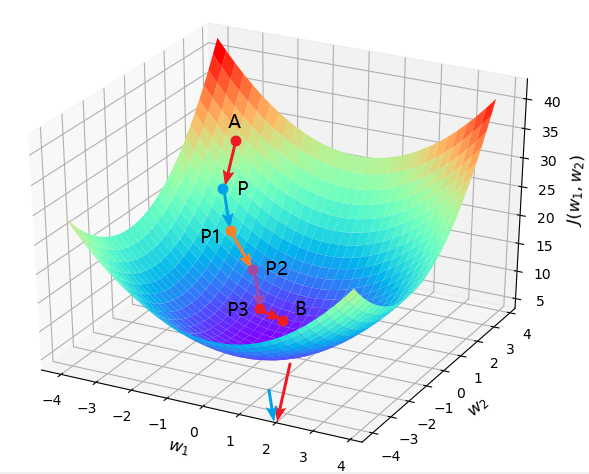#### 2.3 代码示例

xxxxxxxxxx271def cost_function(w1, w2):2    J = w1 ** 2 + w2 ** 2 + 2 * w2 + 53    return J4def compute_gradient(w1, w2):5    return [2 * w1, 2 * w2 + 2]6​7def gradient_descent():8    w1, w2 = -2, 39    jump_points = [[w1, w2]]10    costs = [cost_function(w1, w2)]11    step = 0.112    print("P:({},{})".format(w1, w2), end=' ')13    for i in range(20):14        gradients = compute_gradient(w1, w2)15        w1 = w1 - step * gradients16        w2 = w2 - step * gradients17        jump_points.append([w1, w2])18        costs.append(cost_function(w1, w2))19        print("P{}:({},{})".format(i + 1, round(w1, 3), round(w2, 3)), end=' ')20    return jump_points, costs21​22if __name__ == '__main__':23    jump_points, costs = gradient_descent()24    plot_surface_and_jump_points(jump_points, costs)25    26# 部分结果：P:(-2,3) P1:(-1.6,2.2) P2:(-1.28,1.56).....P20:(-0.023,-0.954)